Students can download Maths Chapter 3 Algebra Ex 3.17 Questions and Answers, Notes, Samacheer Kalvi 10th Maths Guide Pdf helps you to revise the complete Tamilnadu State Board New Syllabus, helps students complete homework assignments and to score high marks in board exams.

## Tamilnadu Samacheer Kalvi 10th Maths Solutions Chapter 3 Algebra Ex 3.17

Question 1.
If then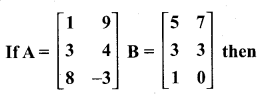verify that (i) A + B = B + A
(ii) A + (-A) = (-A) + A = O.From (1) and (2) we get A + B = B + A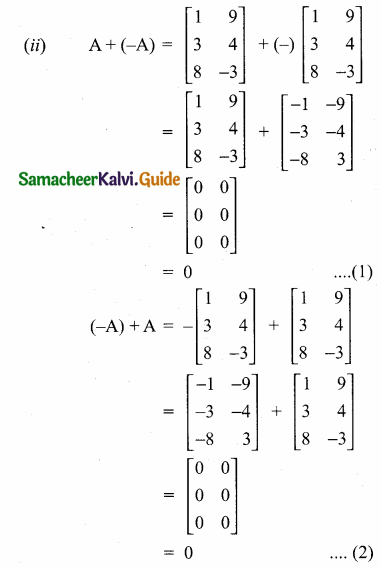From (1) and (2) we get
A + (-A) = (-A) + A = 0Question 2.
If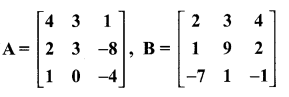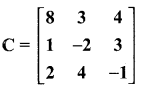then verify that
A + (B + C) = (A + B) + C.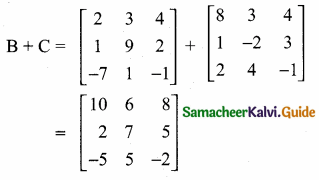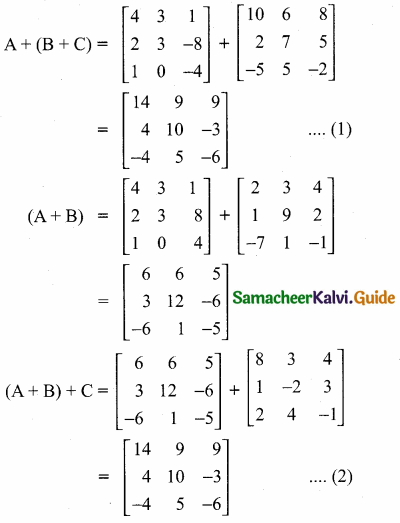From (1) and (2) we get
A + (B + C) = (A + B) + CQuestion 3.
Find X and Y if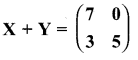and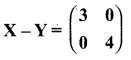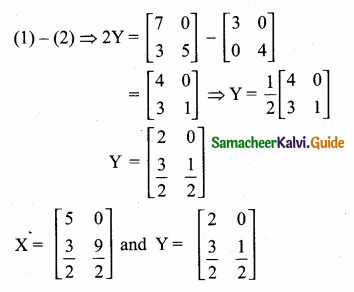Question 4.
If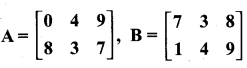find the value of (i) B – 5A (ii) 3A – 9B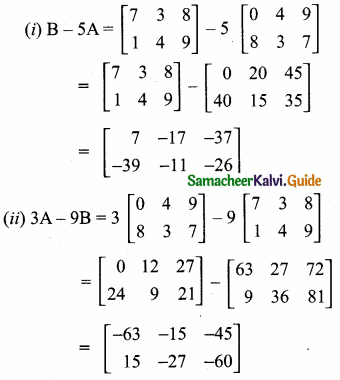Question 5.
Find the values of x, y, z if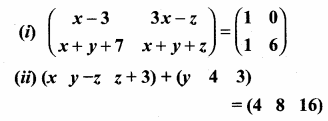(i) x – 3 = 1 ⇒ x = 1 + 3 ⇒ x = 4
3x – z = 0 (substitute the value of x)
3(4) – z = 0
12 – z = 0
∴ z = 12
x + y + z = 6
4 + y + 12 = 0
y + 16 = 6
y = 6- 16
∴ y = -10
The value of x = 4, y = -10 and z = 12

(ii) [x y – z z + 3] + [y 4 3] = [4 8 16]
x + y = 4 ….(1)
y – z + 4 = 8
Substitute the value
of z in (2)
(2) ⇒ y – 10 = 4
Substitute the value of y in (1)
z + 3 + 3 = 16
z + 6 = 16
z = 16 – 16 = 10
y = 14
(1) ⇒ x + 14 = 4
x – 4 – 14 = -10
The value of x = -10, y = 14 and z = 10Question 6.
Find x and y if x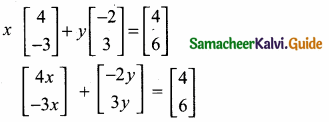4x – 2y = 4
(1) ⇒ 2x – y = 2
(2) ⇒ 3x – y = 2
– x + y = 2Substitute the value of x = 4 in (2)
– 4 + y = 2
y = 2 + 4 = 6
The value of x = 4 and y = 6Question 7.
Find the non-zero values of x satisfying the matrix equation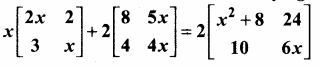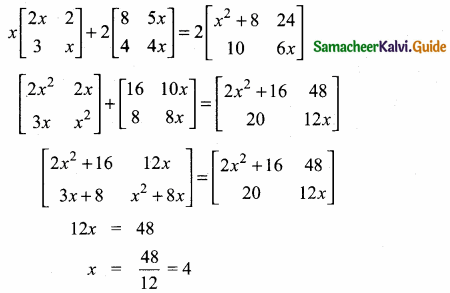The value of x = 4Question 8.
Solve for x,y :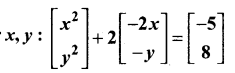x2 – 4x = -5
x2 – 4x + 5 = 0
(x – 5) (x + 1) = 0
x – 5 = 0 or x + 1 = 0
x = 5 or x = – 1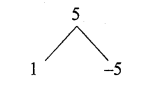The value of x = -1 and 5
y2 – 2y = 8
y2 – 2y – 8 = 0
(y – 4) (y + 2) = 0
y – 4 = 0 or y + 2 = 0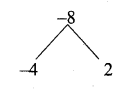y = 4 or y = -2
The value of y = -2 and 4